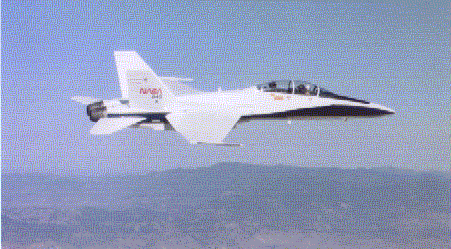PRINT THIS ANSWER SHEET TO FILL OUT AND TURN IN.

NAME_________________________________ CLASS___________________ DATE____________

## Lift Equation Worksheet

1. Identify each letter in the lift equation and list acceptable units for each quantity.

L = __________________________________

Cl = __________________________________

rho = __________________________________

V = __________________________________

A = __________________________________

2.  Open the web page (http://en.wikipedia.org/wiki/Boeing_747), or use a search engine and look up the Boeing 747. What is its wing area? ____________________

3. If the Boeing 747 above is cruising at 940 km/h, the density of the air is 1.20 g/L, and the lift coefficient is 1, what is the lift ? __________________

4. Use the Web page (http://en.wikipedia.org/wiki/Boeing_777), or a search engine to find the Boeing 777. What is its wing area? ___________

5. If the Boeing 777 is cruising at the same velocity under the same conditions as the 747 above, what is its lift? __________________

6. Look up the DC-8 at (http://en.wikipedia.org/wiki/DC-8), or use a search engine. . What is its wing area? ___________

7. What is the DC-8's cruising velocity? _________________

8. If the air density is 1.26 g/L and the lift coefficient is 1.5, what is the lift of the DC-8? _________________

9. Find the F/A-18A Hornet at (http://en.wikipedia.org/wiki/F-18), or use a search engine. What is its wing area? ___________10. What is the F/A-18A's lift while it flies at 700 mph, the air density is 1.31 g/L, and the lift coefficient is 1.8? _________________